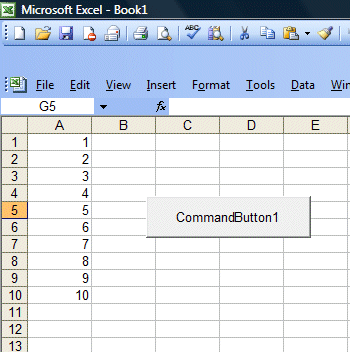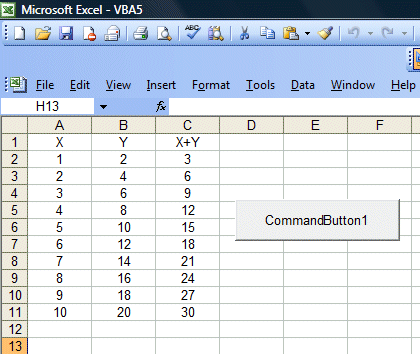Lesson 15: Do...Loop and While...Wend Loop

In the previous lesson, you have learned how to use the For........Next loop. In this lesson,you will learn how to work with two more types of loops, the Do Loop and the While...Wend loop.

15.1 Do Loop

There are four ways you can use the Do Loop as show  below:

i) Do...........Loop While

(ii) Do until.............Loop

(iii) Do while............Loop

(iv) Do............Loop until

Example 15.1 Arranging numbers in ascending order

In this example, the program will keep on adding 1 to the preceding counter value as long as the counter value is less than 10. It displays 1 in cells(1,1), 2 in cells(2,1)….. until 10 in cells (10,1).

Private Sub CommandButton1_Click()

Dim counter As Integer
Do
counter = counter + 1
Cells(counter, 1) = counter
Loop While counter < 10

End Sub

The output is shown in Figure 15.1 on the rightExample 15.2 Arranging numbers in descending order

In this example, the program will keep on adding 1 to the preceding counter value until  the counter value reaches 10. It displays 10 in cells(1,1), 9  in cells(2,1)….. until 1 in cells (10,1).

Private Sub CommandButton1_Click()

Dim counter As Integer
Do Until counter = 10
counter = counter + 1
Cells(counter, 1) = 11 - counter
Loop

End Sub

The output is shown in Figure 15.2 on the rightExamle 15.3

In this example, the program will display the values of X in cells(1,1) to cells(11,1). The value of Y is X2 and the values are display in column 2, i.e. from cells(2,1) to cells(2,11). Finally, it shows the values of X+Y in column 3, i.e. from cells(3,1) to cells(3,11)

Private Sub CommandButton1_Click()
Dim counter , sum As Integer

'To set the alignment to center
Range("A1:C11").Select
With Selection
.HorizontalAlignment = xlCenter
End With

Cells(1, 1) = "X"
Cells(1, 2) = "Y"
Cells(1, 3) = "X+Y"

Do While counter < 10
counter = counter + 1
Cells(counter + 1, 1) = counter
Cells(counter + 1, 2) = counter * 2
sum = Cells(counter + 1, 1) + Cells(counter + 1, 2)
Cells(counter + 1, 3) = sum
Loop
End Sub

The output is shown in Figure 15.3 on the right15.2 While...Wend Loop

The structure of a While...Wend Loop is very similar to the Do Loop. it takes the following form:

While condition
Statements
Wend

Example 15.4

In this example, we add a list box to display a series of numbers and the sum of those numbers. The process of displaying and adding the numbers starting from n=0 till n=19. The process stops when n=20.

Private Sub CommandButton1_Click()
ListBox1.Clear
Dim sum, n As Integer
While n <> 20
n = n + 1
sum = sum + n
ListBox1.AddItem (n & vbTab & sum)
Cells(n + 1, 2) = n
Cells(n + 1, 3) = sum
Wend
End Sub

The output is shown in the Figure 15.4 below>